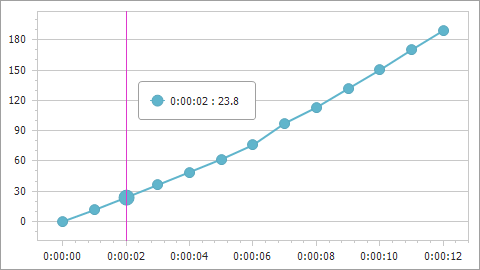# ChartCalculatedFieldType Enum

Lists a calculated field’s returned value types.

Namespace: DevExpress.XtraCharts

Assembly: DevExpress.XtraCharts.v22.2.dll

NuGet Package: DevExpress.Charts

## Declaration

``````[ResourceFinder(typeof(XtraChartsResFinder))]
public enum ChartCalculatedFieldType``````

## Members

Name Description
`None`

Indicates that the field’s type is undefined and determined based on the returned object during chart initialization.

`String`

Indicates that the field returns a string value as a sequence of UTF-16 code units (the String type).

`DateTime`

Indicates that the field returns a value expressed as a date and time of day (the DateTime type).

`TimeSpan`

Indicates that the field returns a value as a time interval (the TimeSpan type).

`Byte`

Indicates that the field returns an 8-bit unsigned integer value (the Byte type).

`Int16`

Indicates that the field returns a 16-bit signed integer value (the Int16 type).

`Int32`

Indicates that the field returns a 32-bit signed integer value (the Int32 type).

`Float`

Indicates that the field returns a single-precision floating-point value (the Single type).

`Double`

Indicates that the field returns a double-precision floating-point value (the Double type).

`Decimal`

Indicates that the field returns a decimal value (the Decimal type).

`Boolean`

Indicates that the field returns a Boolean (true or false) value (the Boolean type).

`Guid`

Indicates that the field returns a global unique identifier value (the Guid type).

## Related API Members

The following properties accept/return ChartCalculatedFieldType values:

Library Related API Members
Cross-Platform Class Library ChartCalculatedField.FieldType
WinForms Controls ChartCalculatedFieldModel.FieldType

## Example

The following example shows how to create a calculated field and then use this field as a data source for a series. The field’s values are calculated by the following expression: [Time.Seconds] * [Velocity].``````using DevExpress.XtraCharts;
using System;
using System.Collections.Generic;
using System.Windows.Forms;

namespace CalculatedFieldExample {
public partial class Form1 : Form {
//...
private void Form1_Load(object sender, EventArgs e) {
chartControl1.DataSource = GetDataPoints();

ChartCalculatedField calcField = new ChartCalculatedField();
calcField.Expression = "[Time.Seconds] * [Velocity]";
calcField.FieldType = ChartCalculatedFieldType.Double;
calcField.Name = "Displacement";

Series series = new Series("series", ViewType.Line);
series.ArgumentDataMember = "Time";
XYDiagram diagram = chartControl1.Diagram as XYDiagram;
diagram.AxisX.TimeSpanScaleOptions.MeasureUnit = TimeSpanMeasureUnit.Second;
diagram.AxisY.WholeRange.AlwaysShowZeroLevel = false;
}
public List<DataPoint> GetDataPoints() {
List<DataPoint> dataPoints = new List<DataPoint>() {
new DataPoint (new TimeSpan(0, 0, 0), 10),
new DataPoint (new TimeSpan(0, 0, 1), 11.46),
new DataPoint (new TimeSpan(0, 0, 2), 11.90),
//...
// Other data points here.
// ...
new DataPoint (new TimeSpan(0, 0, 12), 15.756)
};
return dataPoints;
}
}
public class DataPoint {
public TimeSpan Time { get; set; }
public double Velocity { get; set; }
public DataPoint(TimeSpan time, double velocity) {
this.Time = time;
this.Velocity = velocity;
}
}
}
``````